DraftKings Championship Odds
+1000
10 to 1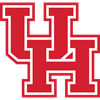Houston
6.1% implied probability

+1000
10 to 1Gonzaga
6.1% implied probability

+1100
11 to 1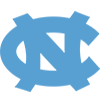North Carolina
5.6% implied probability

+1200
12 to 1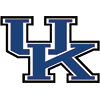Kentucky
5.2% implied probability

+1200
12 to 1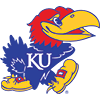Kansas
5.2% implied probability

+1300
13 to 1Duke
4.8% implied probability

+1400
14 to 1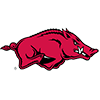Arkansas
4.5% implied probability

+1500
15 to 1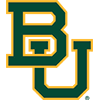Baylor
4.2% implied probability

+1600
16 to 1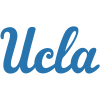UCLA
4.0% implied probability

+2000
20 to 1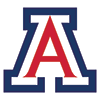Arizona
3.2% implied probability

+2500
25 to 1Creighton
2.6% implied probability

+2500
25 to 1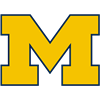Michigan
2.6% implied probability

+3000
30 to 1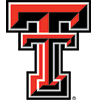Texas Tech
2.2% implied probability

+3500
35 to 1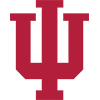Indiana
1.9% implied probability

+3500
35 to 1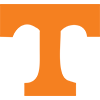Tennessee
1.9% implied probability

+4000
40 to 1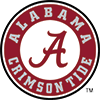Alabama
1.6% implied probability

+4000
40 to 1Villanova
1.6% implied probability

+4000
40 to 1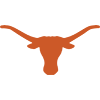Texas
1.6% implied probability

+4500
45 to 1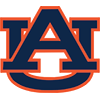Auburn
1.5% implied probability

+4500
45 to 1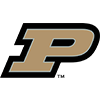Purdue
1.5% implied probability

+5000
50 to 1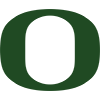Oregon
1.3% implied probability

+6000
60 to 1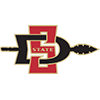San Diego State
1.1% implied probability

+6000
60 to 1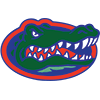Florida
1.1% implied probability

+6000
60 to 1Virginia
1.1% implied probability

+7000
70 to 1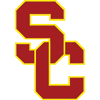USC
1.0% implied probability

+7000
70 to 1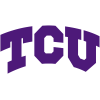TCU
1.0% implied probability

+8000
80 to 1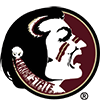Florida State
0.8% implied probability

+8000
80 to 1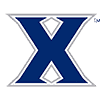Xavier
0.8% implied probability

+8000
80 to 1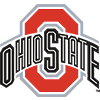Ohio State
0.8% implied probability

+8000
80 to 1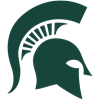Michigan State
0.8% implied probability

+8000
80 to 1Dayton
0.8% implied probability

+9000
90 to 1Connecticut
0.7% implied probability

+10000
100 to 1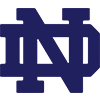Notre Dame
0.7% implied probability

+10000
100 to 1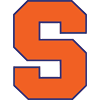Syracuse
0.7% implied probability

+10000
100 to 1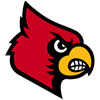Louisville
0.7% implied probability

+10000
100 to 1Wisconsin
0.7% implied probability

+10000
100 to 1Saint Louis
0.7% implied probability

+10000
100 to 1Memphis
0.7% implied probability

+10000
100 to 1Seton Hall
0.7% implied probability

+11000
110 to 1Providence
0.6% implied probability

+12000
120 to 1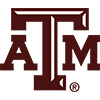Texas A&M
0.6% implied probability

+12000
120 to 1Iowa
0.6% implied probability

+12000
120 to 1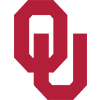Oklahoma
0.6% implied probability

+12000
120 to 1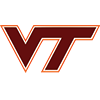Virginia Tech
0.6% implied probability

+12000
120 to 1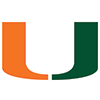Miami
0.6% implied probability

+14000
140 to 1Colorado State
0.5% implied probability

+15000
150 to 1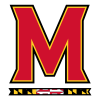Maryland
0.4% implied probability

+15000
150 to 1St. Mary's (CAL)
0.4% implied probability

+15000
150 to 1Davidson
0.4% implied probability

+15000
150 to 1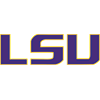Louisiana State
0.4% implied probability

+16000
160 to 1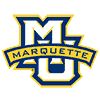Marquette
0.4% implied probability

+16000
160 to 1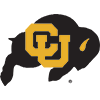Colorado
0.4% implied probability

+20000
200 to 1Arizona State
0.3% implied probability

+20000
200 to 1VCU
0.3% implied probability

+20000
200 to 1West Virginia
0.3% implied probability

+20000
200 to 1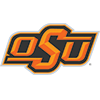Oklahoma State
0.3% implied probability

+20000
200 to 1Wyoming
0.3% implied probability

+20000
200 to 1Butler
0.3% implied probability

+20000
200 to 1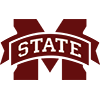Mississippi State
0.3% implied probability

+20000
200 to 1Kansas State
0.3% implied probability

+20000
200 to 1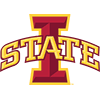Iowa State
0.3% implied probability

+25000
250 to 1St. Bonaventure
0.3% implied probability

+25000
250 to 1Wake Forest
0.3% implied probability

+25000
250 to 1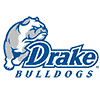Drake
0.3% implied probability

+25000
250 to 1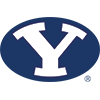BYU
0.3% implied probability

+30000
300 to 1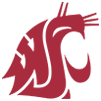Washington State
0.2% implied probability

+30000
300 to 1Wichita State
0.2% implied probability

+30000
300 to 1Belmont
0.2% implied probability

+30000
300 to 1Vanderbilt
0.2% implied probability

+30000
300 to 1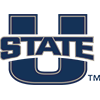Utah State
0.2% implied probability

+35000
350 to 1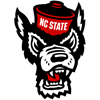North Carolina State
0.2% implied probability

+40000
400 to 1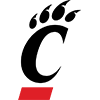Cincinnati
0.2% implied probability

+40000
400 to 1Clemson
0.2% implied probability

+40000
400 to 1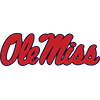Mississippi
0.2% implied probability

+40000
400 to 1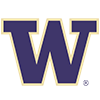Washington
0.2% implied probability

+40000
400 to 1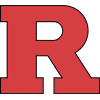Rutgers
0.2% implied probability

+40000
400 to 1Boise State
0.2% implied probability

+40000
400 to 1St. John's
0.2% implied probability

+40000
400 to 1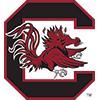South Carolina
0.2% implied probability

+40000
400 to 1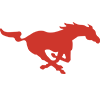SMU
0.2% implied probability

+40000
400 to 1Missouri
0.2% implied probability

+50000
500 to 1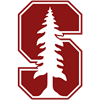Stanford
0.1% implied probability

+50000
500 to 1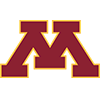Minnesota
0.1% implied probability

+50000
500 to 1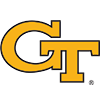Georgia Tech
0.1% implied probability

+50000
500 to 1Richmond
0.1% implied probability

+50000
500 to 1Utah
0.1% implied probability

+50000
500 to 1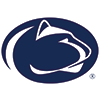Penn State
0.1% implied probability

+50000
500 to 1Georgetown
0.1% implied probability

+50000
500 to 1San Francisco
0.1% implied probability

+60000
600 to 1Boston College
0.1% implied probability

+60000
600 to 1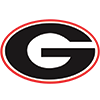Georgia
0.1% implied probability

+60000
600 to 1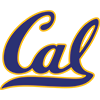California
0.1% implied probability

+60000
600 to 1Fresno State
0.1% implied probability

+60000
600 to 1Murray State
0.1% implied probability

+60000
600 to 1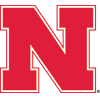Nebraska
0.1% implied probability

+60000
600 to 1Oregon State
0.1% implied probability

+60000
600 to 1Pittsburgh
0.1% implied probability

+60000
600 to 1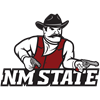New Mexico State
0.1% implied probability